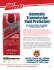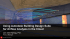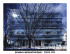# Introduction to Computational Fluid Dynamics

## Transcription

Introduction to Computational Fluid Dynamics
```Introduction to Computational Fluid Dynamics
Instructor: Dmitri Kuzmin
Institute of Applied Mathematics
University of Dortmund
[email protected]
http://www.featflow.de
Fluid (gas and liquid) flows are governed by partial differential equations which
represent conservation laws for the mass, momentum, and energy.
Computational Fluid Dynamics (CFD) is the art of replacing such PDE systems
by a set of algebraic equations which can be solved using digital computers.
http://www.mathematik.uni-dortmund.de/∼kuzmin/cfdintro/cfd.html
What is fluid flow?
Fluid flows encountered in everyday life include
• meteorological phenomena (rain, wind, hurricanes, floods, fires)
• environmental hazards (air pollution, transport of contaminants)
• heating, ventilation and air conditioning of buildings, cars etc.
• combustion in automobile engines and other propulsion systems
• interaction of various objects with the surrounding air/water
• complex flows in furnaces, heat exchangers, chemical reactors etc.
• processes in human body (blood flow, breathing, drinking . . . )
• and so on and so forth
What is CFD?
Computational Fluid Dynamics (CFD) provides a qualitative (and
sometimes even quantitative) prediction of fluid flows by means of
• mathematical modeling (partial differential equations)
• numerical methods (discretization and solution techniques)
• software tools (solvers, pre- and postprocessing utilities)
CFD enables scientists and engineers to perform ‘numerical experiments’
(i.e. computer simulations) in a ‘virtual flow laboratory’
real experiment
CFD simulation
Why use CFD?
Numerical simulations of fluid flow (will) enable
• architects to design comfortable and safe living environments
• designers of vehicles to improve the aerodynamic characteristics
• chemical engineers to maximize the yield from their equipment
• petroleum engineers to devise optimal oil recovery strategies
• surgeons to cure arterial diseases (computational hemodynamics)
• meteorologists to forecast the weather and warn of natural disasters
• safety experts to reduce health risks from radiation and other hazards
• military organizations to develop weapons and estimate the damage
• CFD practitioners to make big bucks by selling colorful pictures :-)
Examples of CFD applications
Aerodynamic shape design
Examples of CFD applications
CFD simulations by Löhner et al.
Examples of CFD applications
Smoke plume from an oil fire in Baghdad
CFD simulation by Patnaik et al.
Experiments vs. Simulations
CFD gives an insight into flow patterns that are difficult, expensive or impossible
to study using traditional (experimental) techniques
Experiments
Simulations
Quantitative description of flow
phenomena using measurements
Quantitative prediction of flow
phenomena using CFD software
• for one quantity at a time
• for all desired quantities
• at a limited number of points
and time instants
• with high resolution in
space and time
• for a laboratory-scale model
• for the actual flow domain
• for a limited range of problems
and operating conditions
• for virtually any problem and
realistic operating conditions
Error sources: measurement errors,
flow disturbances by the probes
Error sources: modeling, discretization, iteration, implementation
Experiments vs. Simulations
As a rule, CFD does not replace the measurements completely but the amount
of experimentation and the overall cost can be significantly reduced.
Experiments
•
•
•
•
expensive
slow
sequential
single-purpose
Simulations
•
•
•
•
cheap(er)
fast(er)
parallel
multiple-purpose
Equipment and personnel
are difficult to transport
CFD software is portable,
easy to use and modify
The results of a CFD simulation are never 100% reliable because
• the input data may involve too much guessing or imprecision
• the mathematical model of the problem at hand may be inadequate
• the accuracy of the results is limited by the available computing power
Fluid characteristics
Macroscopic properties
Classification of fluid flows
ρ
density
viscous
inviscid
µ
viscosity
compressible
incompressible
p
pressure
T
temperature
laminar
turbulent
v
velocity
single-phase
multiphase
The reliability of CFD simulations is greater
• for laminar/slow flows than for turbulent/fast ones
• for single-phase flows than for multi-phase flows
• for chemically inert systems than for reactive flows
How does CFD make predictions?
CFD uses a computer to solve the mathematical equations for the problem
at hand. The main components of a CFD design cycle are as follows:
• the human being (analyst) who states the problem to be solved
• scientific knowledge (models, methods) expressed mathematically
• the computer code (software) which embodies this knowledge and
provides detailed instructions (algorithms) for
• the computer hardware which performs the actual calculations
• the human being who inspects and interprets the simulation results
CFD is a highly interdisciplinary research area which lies at the interface of
physics, applied mathematics, and computer science
CFD analysis process
1.
Problem statement
2.
Mathematical model
IBVP = PDE + IC + BC
3.
Mesh generation
nodes/cells, time instants
4.
Space discretization
coupled ODE/DAE systems
5.
Time discretization
algebraic system Ax = b
6.
Iterative solver
discrete function values
7.
CFD software
implementation, debugging
8.
Simulation run
parameters, stopping criteria
9.
Postprocessing
visualization, analysis of data
Verification
10.
Problem statement
• What is known about the flow problem to be dealt with?
• What physical phenomena need to be taken into account?
• What is the geometry of the domain and operating conditions?
• Are there any internal obstacles or free surfaces/interfaces?
• What is the objective of the CFD analysis to be performed?
– computation of integral quantities (lift, drag, yield)
– snapshots of field data for velocities, concentrations etc.
– shape optimization aimed at an improved performance
• What is the easiest/cheapest/fastest way to achieve the goal?
Mathematical model
1. Choose a suitable flow model (viewpoint) and reference frame.
2. Identify the forces which cause and influence the fluid motion.
3. Define the computational domain in which to solve the problem.
4. Formulate conservation laws for the mass, momentum, and energy.
5. Simplify the governing equations to reduce the computational effort:
• use available information about the prevailing flow regime
• check for symmetries and predominant flow directions (1D/2D)
• neglect the terms which have little or no influence on the results
• model the effect of small-scale fluctuations that cannot be captured
• incorporate a priori knowledge (measurement data, CFD results)
6. Add constituitive relations and specify initial/boundary conditions.
Discretization process
The PDE system is transformed into a set of algebraic equations
1. Mesh generation (decomposition into cells/elements)
• structured or unstructured, triangular or quadrilateral?
• mesh size, adaptive refinement in ‘interesting’ flow regions
2. Space discretization (approximation of spatial derivatives)
• finite differences/volumes/elements
• high- vs. low-order approximations
3. Time discretization (approximation of temporal derivatives)
• explicit vs. implicit schemes, stability constraints
• local time-stepping, adaptive time step control
Iterative solution strategy
The coupled nonlinear algebraic equations must be solved iteratively
• Outer iterations: the coefficients of the discrete problem are updated using
the solution values from the previous iteration so as to
– get rid of the nonlinearities by a Newton-like method
– solve the governing equations in a segregated fashion
• Inner iterations: the resulting sequence of linear subproblems is typically
solved by an iterative method (conjugate gradients, multigrid) because
direct solvers (Gaussian elimination) are prohibitively expensive
• Convergence criteria: it is necessary to check the residuals, relative solution
changes and other indicators to make sure that the iterations converge.
As a rule, the algebraic systems to be solved are very large (millions of unknowns)
but sparse, i.e., most of the matrix coefficients are equal to zero.
CFD simulations
The computing times for a flow simulation depend on
• the choice of numerical algorithms and data structures
• linear algebra tools, stopping criteria for iterative solvers
• discretization parameters (mesh quality, mesh size, time step)
• cost per time step and convergence rates for outer iterations
• programming language (most CFD codes are written in Fortran)
• many other things (hardware, vectorization, parallelization etc.)
The quality of simulation results depends on
• the mathematical model and underlying assumptions
• approximation type, stability of the numerical scheme
• mesh, time step, error indicators, stopping criteria . . .
Postprocessing and analysis
Postprocessing of the simulation results is performed in order to
extract the desired information from the computed flow field
• calculation of derived quantities (streamfunction, vorticity)
• calculation of integral parameters (lift, drag, total mass)
• visualization (representation of numbers as images)
– 1D data: function values connected by straight lines
– 2D data: streamlines, contour levels, color diagrams
– 3D data: cutlines, cutplanes, isosurfaces, isovolumes
– arrow plots, particle tracing, animations . . .
• Systematic data analysis by means of statistical tools
• Debugging, verification, and validation of the CFD model
Uncertainty and error
Whether or not the results of a CFD simulation can be trusted depends on the
degree of uncertainty and on the cumulative effect of various errors
• Uncertainty is defined as a potential deficiency due to the lack of knowledge
(turbulence modeling is a classical example)
• Error is defined as a recognizable deficiency due to other reasons
– Acknowledged errors have certain mechanisms for identifying, estimating
and possibly eliminating or at least alleviating them
– Unacknowledged errors have no standard procedures for detecting them
and may remain undiscovered causing a lot of harm
– Local errors refer to solution errors at a single grid point or cell
– Global errors refer to solution errors over the entire flow domain
Local errors contribute to the global error and may move throughout the grid.
Classification of errors
Acknowledged errors
• Physical modeling error due to uncertainty and deliberate simplifications
• Discretization error ← approximation of PDEs by algebraic equations
– spatial discretization error due to a finite grid resolution
– temporal discretization error due to a finite time step size
• Iterative convergence error which depends on the stopping criteria
• Round-off errors due to the finite precision of computer arithmetic
Unacknowledged errors
• Computer programming error: “bugs” in coding and logical mistakes
• Usage error: wrong parameter values, models or boundary conditions
Awareness of these error sources and an ability to control or preclude the
error are important prerequisites for developing and using CFD software
Verification of CFD codes
Verification amounts to looking for errors in the implementation of the models
(loosely speaking, the question is: “are we solving the equations right”?)
• Examine the computer programming by visually checking the source code,
documenting it and testing the underlying subprograms individually
• Examine iterative convergence by monitoring the residuals, relative changes
of integral quantities and checking if the prescribed tolerance is attained
• Examine consistency (check if relevant conservation principles are satisfied)
• Examine grid convergence: as the mesh and/or and the time step are
refined, the spatial and temporal discretization errors, respectively, should
asymptotically approach zero (in the absence of round-off errors)
• Compare the computational results with analytical and numerical solutions
for standard benchmark configurations (representative test cases)
Validation of CFD models
Validation amounts to checking if the model itself is adequate for practical purposes
(loosely speaking, the question is: “are we solving the right equations”?)
• Verify the code to make sure that the numerical solutions are correct.
• Compare the results with available experimental data (making a provision for
measurement errors) to check if the reality is represented accurately enough.
• Perform sensitivity analysis and a parametric study to assess the inherent
uncertainty due to the insufficient understanding of physical processes.
• Try using different models, geometry, and initial/boundary conditions.
• Report the findings, document model limitations and parameter settings.
The goal of verification and validation is to ensure that the CFD code produces
reasonable results for a certain range of flow problems.
Available CFD software
ANSYS CFX
http://www.ansys.com
commercial
FLUENT
http://www.fluent.com
commercial
STAR-CD
commercial
FEMLAB
http://www.comsol.com
commercial
FEATFLOW
http://www.featflow.de
open-source
• As of now, CFD software is not yet at the level where it can be blindly used by
designers or analysts without a basic knowledge of the underlying numerics.
• Experience with numerical solution of simple ‘toy problems’ makes it easier to
analyze strange looking simulation results and identify the source of troubles.
• New mathematical models (e.g., population balance equations for disperse
systems) require modification of existing / development of new CFD tools.
Structure of the course
1. Introduction, flow models.
2. Equations of fluid mechanics.
3. Finite Difference Method.
4. Finite Volume Method.
5. Finite Element Method.
6. Implementation of FEM.
7. Time-stepping techniques.
8. Properties of numerical methods.
9. Taylor-Galerkin schemes for pure convection.
10. Operator-splitting / fractional step methods.
11. MPSC techniques / Navier-Stokes equations.
12. Algebraic flux correction / Euler equations.
Literature
1. CFD-Wiki
http://www.cfd-online.com/Wiki/Main Page
2. J. H. Ferziger and M. Peric, Computational Methods for Fluid Dynamics.
Springer, 1996.
3. C. Hirsch, Numerical Computation of Internal and External Flows. Vol. I
and II. John Wiley & Sons, Chichester, 1990.
4. P. Wesseling, Principles of Computational Fluid Dynamics. Springer, 2001.
5. C. Cuvelier, A. Segal and A. A. van Steenhoven, Finite Element Methods and
Navier-Stokes Equations. Kluwer, 1986.
6. S. Turek, Efficient Solvers for Incompressible Flow Problems: An Algorithmic
and Computational Approach, LNCSE 6, Springer, 1999.
7. R. Löhner, Applied CFD Techniques: An Introduction Based on Finite Element
Methods. John Wiley & Sons, 2001.
8. J. Donea and A. Huerta, Finite Element Methods for Flow Problems. John
Wiley & Sons, 2003.
```

### (ColPro) Project Arrangement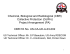### Brochure - MatsyaTeknik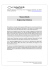### Yucaipa-Calimesa Joint Unified School District Community Facilities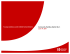### CCTech Consultancy Services Brochure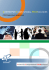### 2016 Spring Conference Agenda April 28-29, 2016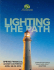### Counselling for depression CfD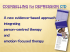### insideswg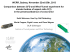### joint exercise of powers agreement creating the south lake tahoe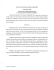### Fluid flow simulations in the undergraduate fluids class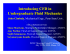### Presentation - CFD4Aircraft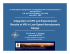### Umbria Region Forecasting/Decision Support for Hydraulic Risk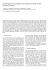### - BIO Web of Conferences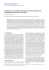### Cooperative project for CFD prediction of pedestrian wind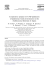### exhaust systems project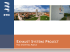### On approaches to couple energy simulation and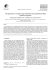### evaluation of numerical methods for turbomachines based on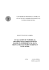### 3356 UK CFD Text 20pp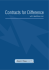### Polynomial sample transmutations of the Normal distribution with an ∗ Unai Ansejo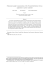### Community Facilities District No. 2004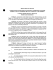### Automatic Transmission Fluid Protectant